# ISEE Middle Level Math : How to find median

## Example Questions

### Example Question #81 : How To Find Median

Find the median in this set of numbers: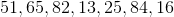Explanation:

To solve, order the numbers from least to greatest: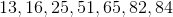Then, identify the middle number: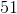Answer: The median is### Example Question #82 : How To Find Median

Use the following data set to answer the question: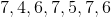Find the median.Explanation:

To find the median of a data set, we must first arrange the set in ascending order. And then we must locate the number in the middle or center of the set.

So, firstly, we need to arrange them from smallest to largest (ascending order). So, in the data setwe will arrange them from smallest to largest.  So,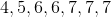Now, we will locate the number in the middle or center of the set.  We can see the numberis in the center of the set.

Therefore,is the median of the data set.

### Example Question #83 : How To Find Median

Find the median in this set of numbers: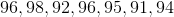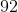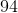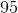Explanation:

To solve, order the numbers from least to greatest: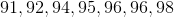Then, find the middle number:Answer: The median is### Example Question #84 : How To Find Median

Subtract the median from the mode in this set of numbers: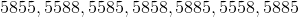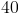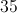Explanation:

First, order the numbers from least to greatest: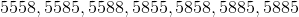Find the median—the middle number: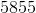Now, find the mode—the most recurring number: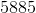Finally, subtract the median from the mode: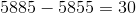### Example Question #85 : How To Find Median

Subtract the range from the median in this set of numbers: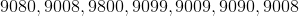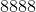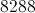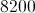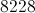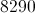Explanation:

First, order the numbers from least to greatest: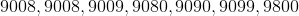In order to find the range, subtract the smallest number from the greatest: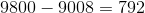Now, find the median by identifying the middle number: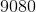Finally, subtract the range from the median: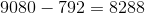### Example Question #86 : How To Find Median

Find the median in this set of numbers: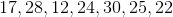Explanation:

First, order the umbers from least to greatest: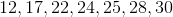Then identify the middle number:Answer:### Example Question #87 : How To Find Median

Find the median in this set of numbers: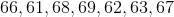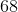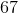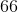Explanation:

First, order the numbers from least to greatest: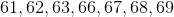Then, identify the middle number:Answer:### Example Question #88 : How To Find Median

What is the median of the following data set?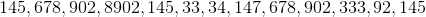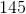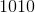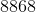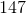Explanation:

What is the median of the following data set?First, put the data set in increasing order: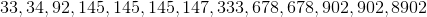Now, our median will simply be the middle term. In this case, it is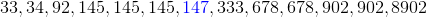It is 147, because there are six terms on either side of 147, making it the middle value

### Example Question #89 : How To Find Median

Use the following data set to answer the question.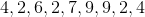Find the median.Explanation:

To find the median of a data set, we will first arrange the numbers in ascending order (from smallest to largest) and then we will locate the number in the center or the middle.

So, given the setwe will arrange them in ascending order.  We get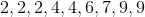Now, we will locate the number that is in the center or the middle.  We can see that it is.

Therefore, the median of the data set is.

### Example Question #90 : How To Find Median

Find the median of the following data set: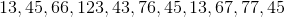Explanation:

Find the median of the following data set:Let's begin by ordering our data set from least to greatest.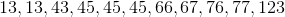Now, our median can be identified by looking at the number or pair of numbers in the middle of our set.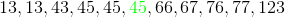In this case, 45 is our median, because it is the middle value. There are 5 numbers to either side of it.

### All ISEE Middle Level Math Resources